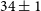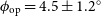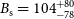Hostname: page-component-546b4f848f-hhr79 Total loading time: 0 Render date: 2023-06-03T12:12:56.351Z Has data issue: false Feature Flags: { "useRatesEcommerce": true } hasContentIssue false

# A broadband radio view of transient jet ejecta in the black hole candidate X-ray binary MAXI J1535–571

Published online by Cambridge University Press:  07 September 2021

*
*Author for correspondence: Jaiverdhan Chauhan, E-mail: j.chauhan@student.curtin.edu.au and J. C. A. Miller-Jones, E-mail: James.Miller-Jones@curtin.edu.au
*Author for correspondence: Jaiverdhan Chauhan, E-mail: j.chauhan@student.curtin.edu.au and J. C. A. Miller-Jones, E-mail: James.Miller-Jones@curtin.edu.au

## Abstract

We present a broadband radio study of the transient jets ejected from the black hole candidate X-ray binary MAXI J1535–571, which underwent a prolonged outburst beginning on 2017 September 2. We monitored MAXI J1535–571 with the Murchison Widefield Array (MWA) at frequencies from 119 to 186 MHz over six epochs from 2017 September 20 to 2017 October 14. The source was quasi-simultaneously observed over the frequency range 0.84–19 GHz by UTMOST (the Upgraded Molonglo Observatory Synthesis Telescope) the Australian Square Kilometre Array Pathfinder (ASKAP), the Australia Telescope Compact Array (ATCA), and the Australian Long Baseline Array (LBA). Using the LBA observations from 2017 September 23, we measured the source size to be$34\pm1$ mas. During the brightest radio flare on 2017 September 21, the source was detected down to 119 MHz by the MWA, and the radio spectrum indicates a turnover between 250 and 500 MHz, which is most likely due to synchrotron self-absorption (SSA). By fitting the radio spectrum with a SSA model and using the LBA size measurement, we determined various physical parameters of the jet knot (identified in ATCA data), including the jet opening angle ($\phi_{\rm op} = 4.5\pm1.2^{\circ}$ ) and the magnetic field strength ($B_{\rm s} = 104^{+80}_{-78}$ mG). Our fitted magnetic field strength agrees reasonably well with that inferred from the standard equipartition approach, suggesting the jet knot to be close to equipartition. Our study highlights the capabilities of the Australian suite of radio telescopes to jointly probe radio jets in black hole X-ray binaries via simultaneous observations over a broad frequency range, and with differing angular resolutions. This suite allows us to determine the physical properties of X-ray binary jets. Finally, our study emphasises the potential contributions that can be made by the low-frequency part of the Square Kilometre Array (SKA-Low) in the study of black hole X-ray binaries.

Type
Research Article
© The Author(s), 2021. Published by Cambridge University Press on behalf of the Astronomical Society of Australia

## Access options

Get access to the full version of this content by using one of the access options below. (Log in options will check for institutional or personal access. Content may require purchase if you do not have access.)

## References

Atri, P., et al. 2020, MNRAS, 493, L81 CrossRefGoogle Scholar
Bailes, M., et al. 2017, PASA, 34, e045 Google Scholar
Barthelmy, S. D., et al. 2005, SSRv, 120, 143 CrossRefGoogle Scholar
Begelman, M. C., Blandford, R. D., & Rees, M. J. 1984, RvMP, 56, 255 CrossRefGoogle Scholar
Belloni, T. M., 2010, States and Transitions in Black Hole Binaries. p. 53, doi: 10.1007/978-3-540-76937-8_3 CrossRefGoogle Scholar
Blandford, R. D., & Königl, A. 1979, ApJ, 232, 34 CrossRefGoogle Scholar
Bright, J. S., et al. 2020, Nature Astronomy, 4, 697 Google Scholar
Callingham, J. R., et al. 2015, ApJ, 809, 168 Google Scholar
Caplan, J., & Deharveng, L. 1986, A&A, 155, 297 Google Scholar
Casella, P., et al. 2010, MNRAS, 404, L21 CrossRefGoogle Scholar
Chandra, P., & Kanekar, N. 2017, ApJ, 846, 111 CrossRefGoogle Scholar
Chauhan, J., et al. 2019a, MNRAS, p. L116 Google Scholar
Chauhan, J., Miller-Jones, J., Anderson, G., Russell, T., Hancock, P., Bahramian, A., Duchesne, S., & Williams, A. 2019b, ATel, 12520, 1 Google Scholar
Corbel, S., et al. 2015, in Advancing Astrophysics with the Square Kilometre Array (AASKA14), 53 (arXiv:1501.04716)Google Scholar
Coriat, M., Fender, R. P., & Dubus, G. 2012, MNRAS, 424, 1991 CrossRefGoogle Scholar
Corral-Santana, J. M., Casares, J., Muñoz-Darias, T., Bauer, F. E., Martínez-Pais, I. G., & Russell, D. M. 2016, A&A, 587, A61 CrossRefGoogle Scholar
Deller, A. T., Tingay, S. J., Bailes, M., & West, C. 2007, PASP, 119, 318 CrossRefGoogle Scholar
Deller, A. T., et al. 2011, PASP, 123, 275 CrossRefGoogle Scholar
Dinçer, T. 2017, ATel 10716, 1 CrossRefGoogle Scholar
Dubus, G., Hameury, J. M., & Lasota, J. P. 2001, A&A, 373, 251 CrossRefGoogle Scholar
Duchesne, S. W., Johnston-Hollitt, M., Zhu, Z., Wayth, R. B., & Line, J. L. B. 2020, PASA, 37, e037 CrossRefGoogle Scholar
Esin, A. A., McClintock, J. E., & Narayan, R., 1997, ApJ, 489, 865 CrossRefGoogle Scholar
Espinasse, M., Carotenuto, F., Tremou, E., Corbel, S., Fender, R., Woudt, P., & Miller-Jones, J. 2021, ATel, 14607, 1 Google Scholar
Fender, R. P. 2001, MNRAS, 322, 31 CrossRefGoogle Scholar
Fender, R. P. 2003, MNRAS, 340, 1353 CrossRefGoogle Scholar
Fender, R. 2006, Jets from X-ray Binaries, 381–419CrossRefGoogle Scholar
Fender, R., & Bright, J. 2019, MNRAS, 489, 4836 Google Scholar
Fender, R., & Gallo, E. 2014, SSRv, 183,323 CrossRefGoogle Scholar
Fender, R. P., Belloni, T. M., & Gallo, E. 2004, MNRAS, 355,1105 CrossRefGoogle Scholar
Fendt, C., & Sheikhnezami, S. 2013, ApJ, 774,12 CrossRefGoogle Scholar
Ferrari, A. 1998, ARA&A, 36, 539 CrossRefGoogle Scholar
Finkbeiner, D. P. 2003, ApJS, 146, 407 CrossRefGoogle Scholar
Frater, R. H., Brooks, J. W., & Whiteoak, J. B. 1992, J. Electric. Electron. Eng. Australia, 12, 103 Google Scholar
Gallo, E., Fender, R., Kaiser, C., Russell, D., Morganti, R., Oosterloo, T., & Heinz, S. 2005, Nature, 436, 819 Google Scholar
Gaustad, J. E., McCullough, P. R., Rosing, W., & Van Buren, D. 2001, PASP, 113, 1326 CrossRefGoogle Scholar
Gehrels, N., et al. 2004, ApJ, 611, 1005 Google Scholar
Gregory, P. C., & Seaquist, E. R. 1974, ApJ, 194, 715 CrossRefGoogle Scholar
Greisen, E. W. 2003, AIPS, the VLA, and the VLBA. p. 109, doi: 10.1007/0-306-48080-8_7 CrossRefGoogle Scholar
Hancock, P. J., Hurley-Walker, N., & White, T. E. 2019, A&C, 27, 23 CrossRefGoogle Scholar
Hannikainen, D. C., Hunstead, R. W., Campbell-Wilson, D., & Sood, R. K. 1998, A&A, 337, 460 Google Scholar
Harmon, B. A., et al. 1995, Nature, 374, 703 Google Scholar
Hjellming, R. M., & Johnston, K. J. 1988, ApJ, 328, 600 CrossRefGoogle Scholar
Hjellming, R. M., & Rupen, M. P. 1995, Nature, 375, 464 Google Scholar
Hotan, A. W., et al. 2014, PASA, 31, e041 Google Scholar
Hotan, A. W., et al. 2021, PASA, 38, e009 Google Scholar
Hurley-Walker, N., & Hancock, P. J. 2018, A&C, 25, 94 CrossRefGoogle Scholar
Hurley-Walker, N., et al., 2017, MNRAS, 464, 1146 Google Scholar
Jauncey, D. L., et al. 1994, in IAU Symposium 158, ed. Robertson, J. G., & Tango, W. J., Vol. 158, Very High Angular Resolution Imaging, 131–134Google Scholar
Kellermann, K. I. 1966, AuJPh, 19, 577 CrossRefGoogle Scholar
Kennea, J. A., Evans, P. A., Beardmore, A. P., Krimm, H. A., Romano, P., Yamaoka, K., Serino, M., & Negoro, H. 2017, ATel, 10700, 1 Google Scholar
Koljonen, K. I. I., McCollough, M. L., Hannikainen, D. C., & Droulans, R. 2013, MNRAS, 429, 1173 CrossRefGoogle Scholar
Koljonen, K. I. I., Maccarone, T., McCollough, M. L., Gurwell, M., Trushkin, S. A., Pooley, G. G., Piano, G., & Tavani, M. 2018, A&A, 612, A27 CrossRefGoogle Scholar
Lasota, J.-P. 2001, New Astron. Rev., 45, 449 Google Scholar
Lasota, J.-P. 2008, New Astron. Rev., 51, 752 Google Scholar
Maccarone, T. J. 2002, MNRAS, 336, 1371 CrossRefGoogle Scholar
Marscher, A. P. 1983, ApJ, 264, 296 CrossRefGoogle Scholar
Matsuoka, M., et al. 2009, PASJ, 61, 999 CrossRefGoogle Scholar
McMullin, J. P., Waters, B., Schiebel, D., Young, W., & Golap, K. 2007, in Astronomical Data Analysis Software and Systems XVI, ed. Shaw, R. A., Hill, F., & Bell, D. J., Astronomical Society of the Pacific Conference Series, Vol. 376, 127 Google Scholar
Mezger, P. G., & Henderson, A. P. 1967, ApJ, 147, 471 CrossRefGoogle Scholar
Miller-Jones, J. C. A., Blundell, K. M., Rupen, M. P., Mioduszewski, A. J., Duffy, P., & Beasley, A. J. 2004, ApJ, 600, 368 CrossRefGoogle Scholar
Miller-Jones, J. C. A., Fender, R. P., & Nakar, E., 2006, MNRAS, 367, 1432 CrossRefGoogle Scholar
Miller-Jones, J. C. A., et al. 2012, MNRAS, 419, L49 Google Scholar
Miller-Jones, J. C. A., et al. 2021, Science, 371, 1046 Google Scholar
Miller, J. M., et al. 2018, ApJ, 860, L28 CrossRefGoogle Scholar
Mirabel, I. F., & Rodríguez, L. F. 1994, Nature, 371, 46 Google Scholar
Murphy, T., Mauch, T., Green, A., Hunstead, R. W., Piestrzynska, B., Kels, A. P., & Sztajer, P. 2007, MNRAS, 382, 382 CrossRefGoogle Scholar
Nakahira, S., et al. 2018, PASJ, 70, 95 Google Scholar
Negoro, H., et al. 2017, ATel, 10699, 1 Google Scholar
Offringa, A. R., de Bruyn, A. G., & Zaroubi, S. 2012, MNRAS, 422, 563 CrossRefGoogle Scholar
Offringa, A. R., et al. 2014, MNRAS, 444, 606 Google Scholar
Offringa, A. R., et al. 2015, PASA, 32,e008 Google Scholar
Osterbrock, D. E., & Ferland, G. J. 2006, Astrophysics of gaseous nebulae and active galactic nucleiGoogle Scholar
Parikh, A. S., Russell, T. D., Wijnands, R., Miller-Jones, J. C. A., Sivako, G. R., & Tetarenko, A. J. 2019, ApJ, 878, L28 CrossRefGoogle Scholar
Partridge, B., López-Caniego, M., Perley, R. A., Stevens, J., Butler, B. J., Rocha, G., Walter, B., & Zacchei, A. 2016, ApJ, 821, 61 CrossRefGoogle Scholar
Plotkin, R. M., et al. 2017, ApJ, 834, 104 CrossRefGoogle Scholar
Preston, R. A., & Team, SHEVE 1993, in Sub-arcsecond Radio Astronomy, ed. Davis, R. J., & Booth, R. S., 428Google Scholar
Preston, R. A., et al. 1989, AJ, 98, 1 Google Scholar
Remillard, R. A., & McClintock, J. E. 2006, ARA&A, 44, 49 CrossRefGoogle Scholar
Rushton, A. P., et al. 2017, MNRAS, 468, 2788 CrossRefGoogle Scholar
Russell, T. D., Soria, R., Miller-Jones, J. C. A., Curran, P. A., Markoff, S., Russell, D. M., & Sivakoff, G. R. 2014, MNRAS, 439, 1390 CrossRefGoogle Scholar
Russell, T. D., Miller-Jones, J. C. A., Sivakoff, G. R., Tetarenko, A. J., & Jacpot Xrb, Collaboration 2017, ATel, 10711, 1 Google Scholar
Russell, T. D., et al. 2019, ApJ, 883, 198 Google Scholar
Russell, T. D., et al. 2020, MNRAS, 498, 5772 Google Scholar
Rybicki, G. B., & Lightman, A. P. 1979, AQ, 3, 199 Google Scholar
Salvatier, J., Wieckiâ, T. V., & Fonnesbeck, C. 2016, PyMC3: Python probabilistic programming framework (ascl:1610.016)Google Scholar
Seaquist, E. R. 1976, ApJ, 207, 88 CrossRefGoogle Scholar
Seaquist, E. R., Gilmore, W., Nelson, G. J., Payten, W. J., & Slee, O. B. 1980, ApJ, 241, 77 CrossRefGoogle Scholar
Seaquist, E. R., Gilmore, W. S., Johnston, K. J., & Grindlay, J. E. 1982, ApJ, 260, 220 CrossRefGoogle Scholar
Shepherd, M. C. 1997, in Astronomical Data Analysis Software and Systems VI, ed. Hunt, G., & Payne, H., Astronomical Society of the Pacific Conference Series, Vol. 125, 77Google Scholar
Stirling, A. M., Spencer, R. E., de la Force, C. J., Garrett, M. A., Fender, R. P., & Ogley, R. N. 2001, MNRAS, 327, 1273 CrossRefGoogle Scholar
Tananbaum, H., Gursky, H., Kellogg, E., Giacconi, R., & Jones, C. 1972, ApJ, 177, L5 CrossRefGoogle Scholar
Tang, J., Yu, W.-F., & Yan, Z. 2011, RAA, 11, 434 CrossRefGoogle Scholar
Tao, L., et al. 2018, MNRAS, 480, 4443 CrossRefGoogle Scholar
Tetarenko, B. E., Sivakoff, G. R., Heinke, C. O., & Gladstone, J. C. 2016, ApJS, 222, 15 CrossRefGoogle Scholar
Tetarenko, A. J., et al. 2017, MNRAS, 469, 3141 CrossRefGoogle Scholar
Tetarenko, A. J., Freeman, P., Rosolowsky, E. W., Miller-Jones, J. C. A., & Sivakoff, G. R. 2018, MNRAS, 475, 448 CrossRefGoogle Scholar
Tetarenko, A. J., Casella, P., Miller-Jones, J. C. A., Sivakoff, G. R., Tetarenko, B. E., Maccarone, T. J., Gandhi, P., & Eikenberry, S. 2019, MNRAS, 484, 2987 CrossRefGoogle Scholar
Tetarenko, A. J., et al. 2021, MNRAS,Google Scholar
Thorne, K. S., & Price, R. H., 1975, ApJ, 195, L101 CrossRefGoogle Scholar
Tingay, S. J., & de Kool, M. 2003, AJ, 126, 723 CrossRefGoogle Scholar
Tingay, S. J., et al. 1995, Nature, 374, 141 Google Scholar
Tingay, S. J., et al. 2013, PASA, 30, e007 Google Scholar
Vadawale, S. V., Rao, A. R., Naik, S., Yadav, J. S., Ishwara-Chandra, C. H., Pramesh Rao, A., & Pooley, G. G. 2003, ApJ, 597, 1023 CrossRefGoogle Scholar
Wayth, R. B., et al. 2018, PASA, 35 Google Scholar
Williams, D., Fender, R., Woudt, P., & Miller-Jones, J. 2019, ATel, 12992, 1 Google Scholar
Wilson, W. E., et al. 2011, MNRAS, 416, 832 Google Scholar
Xu, Y., et al. 2018, ApJ, 852, L34 CrossRefGoogle Scholar
Xu, Y., Harrison, F. A., Tomsick, J. A., Hare, J., Fabian, A. C., & Walton, D. J. 2020, ApJ, 893, 42 CrossRefGoogle Scholar
van Paradijs, J., & Verbunt, F. 1984, in High Energy Transients in AstroPhysics, ed. Woosley, S. E., Institute, American of Physics Conference Series, Vol. 115, 49–62, doi: 10.1063/1.34556 CrossRefGoogle Scholar
van der Laan, H. 1966, Nature, 211, 1131 CrossRefGoogle Scholar